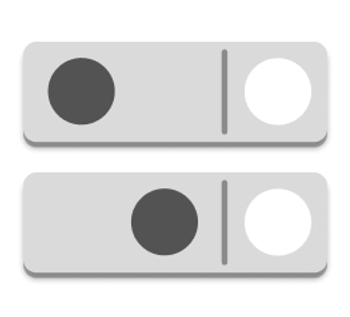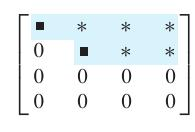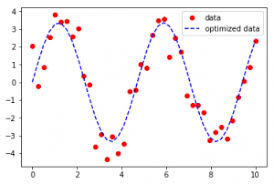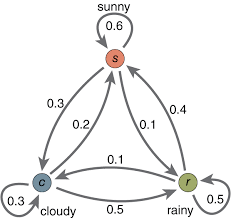Project

For this class you can choose one of the projects below. The aim is to explore a topic of the course in depth. Each project is for a team of 4-6 students. For the first four projects at least two team members must have experience with programming.

Linear Games
see webpage.Only four out of ten high school students feel engaged in class (Gallup, 2015) and half of the students feel bored and tired. Especially concerned by this trend is the subject math. A very recent idea to solve this problem is the gamification of math. Following this approach we developed three computer games that teach high school and college students math in a fun and open setting. Here we focused on Linear Algebra whose mastery is essential for almost all basic sciences. To focus on the algorithmic side instead of complicated calculations we replaced the real numbers by a simpler number system which in these games is represented by pieces on a Go board.
Take a look at these games and read "The math behind these games".

Project 1: Write a version of the game Sweep the board where the input is a random matrix and the player has to row reduce the matrix and then enter a correct solution vector or key. A python version of the original game can be found below.
Project 2: Write a version of game Matrix Labyrinth.
Project 3: Write your own game inspired by Linear Algebra over the field with three numbers.

Resources

Row Reduction AlgorithmRow reduction is an algorithm for solving a system of linear equations. It is usually understood as a sequence of operations performed on the corresponding matrix of coefficients. This method can also be used to find the rank of a matrix, to calculate the determinant of a matrix, and to calculate the inverse of an invertible square matrix. It was known to Chinese mathematicians as early as 179 AD.

Project 4: Describe in detail (with proofs) the row reduction algorithm from class and how it can be used to find the determinant of a matrix. Then write your own program for the row reduction algorithm. The input should be an augmented matrix and the output the set of solutions. Additionally write a program that finds the determinant of a matrix using the row reduction algorithm.

Resources
Lay, D. et al: Linear Algebra and its Applications, Chapter 1, 3.1, 3.2

Curve FittingCurve fitting is the process of constructing a curve, or mathematical function, that has the best fit to a series of data points. Curve fitting can involve either interpolation, where an exact fit to the data is required, or smoothing, in which a smooth function is constructed that approximately fits the data. A related topic is regression analysis, which focuses more on statistical questions concerning curve fitting. The approximating curve can be constructed using a Least Square method from Linear Algebra.

Project 5: Set up your own curve fitting problem and solve it. You could, for example, look at the climate data in Hanover or make a predictor for the result of our final exam based on the midterms using data from older courses.

Resources

• Lay, D. et al: Linear Algebra and its Applications, browse Chapter 6.6, then read through Chapter 6
• Wikipedia - Curve fitting
• Wikipedia - Least Square method
• Data sets available upon request

Flow in NetworksTransitions or flows in networks can be analyzed by writing the information into a matrix. Finding the steady state of the system amounts to finding an eigenvector of this matrix. This method has many applications. This way one can, for example, study exchange rates of currencies, queues or lines of customers arriving at an airport or population growths of certain animal species. The algorithm known as PageRank, which was originally proposed for the internet search engine Google is based on this idea.

Project 6: Read the information below. Then create your own network with flows and find the steady state of the system. Choose a meaningful network and flow (network of friends, sandbox internet ...) and explain your result.

Resources

Requirements
The project should be described in a short report / essay of at least 4 pages. The following topics should be included:
• Introduction
• Description of the project
• Result
• Discussion / Observations
• Bibliography
Additionally, on the second last day of the course each group will present their project in a short 10 min. presentation.

Teams

 Section 1Temperature over the yearsLinear Games - Sweep the board 1Research output vs. alumni employmentScores from Math 22Linear Games - Sweep the board 2City population and CO2 footprinting Section 2US plane trafficTornado trendsEducation vs. life expectancyRow Reduction algorithmDow Jones vs. presidential approvalOKCupid profilesEnergy consumption during the year

Bjoern Muetzel
Last updated November 18, 2019